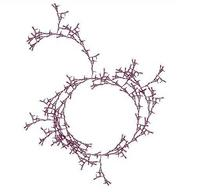University of Virginia, Department of Computer Science CS200: Computer Science, Spring 2003

Notes: Wednesday 12 March 2003

• Friday, 14 March: Problem Set 5: Wahoo! Auctions
• Wednesday, 19 March: Read GEB, Aria with Diverse Variations and Chapter 13. This chapter proves that the halting problem is not decidable, and introduces the Church-Turing Thesis (which we will explore more in Classes 32-36). You will not be assigned to read Chapter XIV, but it goes into more depth on Gödel's proof and it recommended.
• Monday, 31 March: Problem Set 8 team requests. For PS8, you will work in teams of 1-6 people (preferably 3 in most cases) to build a dynamic, database-backed web site. If you want to request your own team, you need to decide as a group to be a team, and send me a single request that you cc: to all your potential team members. In order to increase the chances I honor your request, your email should not only list the team members but should give a brief description of the web site you have agreed as a group to build. Students who do not request teams, will be arbitrarily grouped into teams.

Notes
Procedure: A precise description of a process.

Alrogithm: A procedure that always terminates.

Proof: A proof of S in an axiomatic system is a sequence of strings, T0, T1, ..., Tn where:

• The first string is the axioms
• For all i from 1 to n, Tn is the result of applying one of the inference rules to Tn-1
• Tn is S
Proof Checking Problem

Input: an axiomatic system (a set of axioms and inference rules), a statement S, and a proof P containing n steps of S.

Output: true if P is a valid proof of S; false otherwise.

How much work is a proof checking procedure?

Finite-Length Proof Finding Problem

Input: an axiomatic system (a set of axioms and inference rules), a statement S, n (the maximum number of proof steps)

Output: A valid proof of S with no more then n steps if there is one. If there is no proof of S with <= n steps, unprovable.

How much work is a straightforward proof finding procedure?

n — maximum number of steps
r — number of inference rules

At worst, we can try all possible rules at every step:

Proof Finding Problem

Input: an axiomatic system, a statement S.

Output: If S is true, output is a valid proof. If S is not true, output is false.

Can we write an algorithm to solve the proof finding problem?

Computability

Is there an algorithm (a procedure that always terminates) that solves the problem?

A problem is decidable is there exists an algorithm that can solve the problem for all possible inputs. It is not necessary to know what that algorithm is to say a problem is decidable, only to know that some algorithm to solve it must exist. For example, chess is a decidable problem, even if we do not yet know an algorithm that solves it.

A problem is undecidable is there is no algorithm that can solve the problem. There might be a procedure, but it is not guaranteed to terminate.

Is the proof finding problem decidable?

How can you prove a problem is undecidable?

Halting Problem

Input: a procedure P (described by a Scheme program), and the input to that procedure
Output: true if applying P to input halts (finishes execution), false otherwise.

```(define (halts? procedure input) ...?...)
```
```(define (find-proof S axioms rules)
;; If S is provable, evaluates to a proof of S.
;; Otherwise, evaluates to #f.
(if (halts? find-proof-exhaustive
S axioms rules)
(find-proof-exhaustive S axioms rules)
#f))
```
Where (find-proof-exhaustive S axioms rules) is a procedure that tries all possible proofs starting from the axioms that evaluates to a proof if it finds one, and keeps working if it doesn't.
We can prove the halting problem is undecidable informally by arguing that if we could define halts?, we could use it to define contradict-halts (the input parameter to contradict-halts is not used, but necessary because of how we defined halts?):
```(define (contradict-halts input)
(infinite-loop)
200))
```
But contradict-halts is non-sensical: if it halts, it loops infinitely; if it doesn't halt, it evaluates to 200 and halts. This means something in the program must not exist. I'm pretty sure 200 exists, and we know how to define infinite-loop, and if seems likely to exist (and its worked well for us so far). So, it must be halts? that cannot exist.

Undecidable Problems If solving a problem P would allow us to solve the halting problem, then P is undecidable — there is no solution to P, since we have proved there is no solution to the halting problem!

Why will there never be a perfect virus detection program?

Malicious Code Problem

Input: a procedure P
Output: true if P is would do something bad, false otherwise.

Assume we have a precise definition of what something bad means (for example, format your hard drive).

Is the malicious code problem decidable?

```(define (halts? P input)
(is-virus? `(begin ((vaccinate P) input)
virus-code)))
```
Where (vaccinate P) evaluates to P with all mail commands replaced with print commands (to make sure (is-virus? P input) is false.CS 200: Computer Science Department of Computer Science University of Virginia Circle Fractal by Ramsey Arnaoot and Qi Wang cs200-staff@cs.virginia.edu Using these Materials